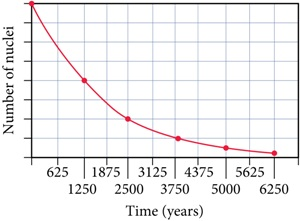# Problem: Consider this graph representing the decay of a radioactive nuclide.What is the half-life of the nuclide?

###### FREE Expert Solution

We’re being asked to determine the half-life of the nuclide using the graph representing the decay of a radioactive nuclide.

Recall that radioactive/nuclear decay of isotopes follows first-order kinetics, and the integrated rate law for first-order reactions is:

where:

[N]t = concentration at time t

k = decay constant

t = time

[N]0 = initial concentration

Also, recall that half-life is the time needed for the amount of a reactant to decrease by 50% or one-half

85% (97 ratings)###### Problem Details

Consider this graph representing the decay of a radioactive nuclide.What is the half-life of the nuclide?

Frequently Asked Questions

What scientific concept do you need to know in order to solve this problem?

Our tutors have indicated that to solve this problem you will need to apply the First Order Half Life concept. You can view video lessons to learn First Order Half Life. Or if you need more First Order Half Life practice, you can also practice First Order Half Life practice problems.

What professor is this problem relevant for?

Based on our data, we think this problem is relevant for Professor Whitney's class at University of North Dakota.